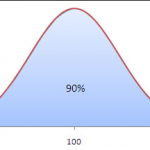# 90 percentile response time

by Swaraj GuptaThe response time value for a transaction below which 90% of the data points (response time values) lie, is called the 90 percentile response time.

In order to obtain the 90 percentile response time value for a transaction, sort all the response time values for that transaction in increasing order. Take the first 90 % transactions out of this set. The response time that has the maximum value in this set is the 90 percentile value of the studied transaction.

If you use Microsoft excel to calculate the 90 percentile value, you can use its PERCENTILE function. The function is used as =PERCENTILE(array,k), where k is the percentile value you want to calculate. For 90 percentile, k would be 0.9.

Example – For a transaction, let’s say there are 10 response time values are available –

1, 2, 3, 4, 5, 6, 7, 8, 9 & 10.

I have sorted these numbers above. If I take out 90 percent response time values out as a separate set, I will get –

1, 2, 3, 4, 5, 6, 7, 8 & 9.

Here 9 is the maximum value and hence is the 90 percentile value of that transaction.

Scenarios in which 90 percentile values can be useful

Scenario 1: When average response time appear to be extremely high and individual data sets seem normal. During some tests, a couple of peaks in response times, skew the average response time numbers and impact the test. In such scenarios, 90 percentile (or other percentile values) are looked and studied and if the percentile value is not high, the average is adjusted accordingly. Thus 90 percentile values can be extremely useful in the result analysis phase of the test cycle.Scenario 2: To understand the spread of response time values. Taking a difference of the 90 percentile value and the average response time value and dividing this difference with the average response time value gives an idea of the spread of different data points. If the ratio is extremely small, it means that average and 90 percentile values are very close to each other and data points are close to each other. However if the ratio is large, it gives the opposite idea.

Having said that std dev is still a better counter to study the spread of data points.

This post is also available in: French